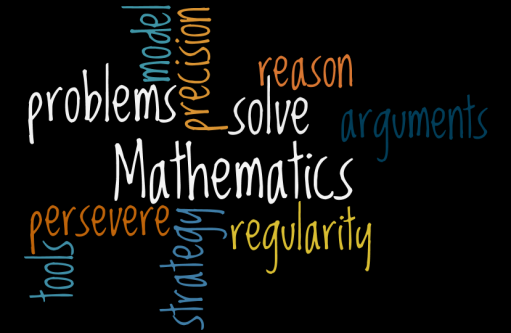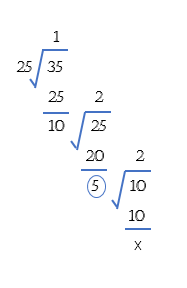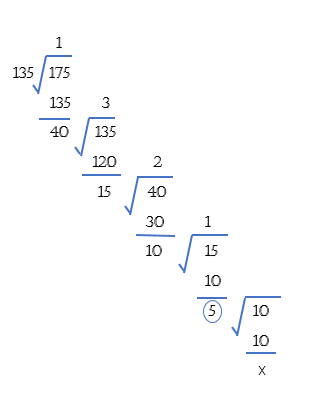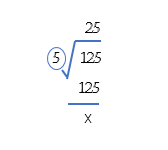# Trick Alert: Fastest way to find HCF of numbers – Exclusively on AEIRO

##### Hello Mathematicians,

Today, I will show you how to find H.C.F (Highest Common Factor) of two or more numbers the quickest way. You won’t find this trick anywhere, this is exclusively available here on Aeiro.Com.

#### What’s H.C.F.?

HCF or Highest Common Factor is the biggest number that can divide each of the numbers in question. You can find HCF of two or more numbers.

Now let’s first see how we usually find HCF of numbers say 25 and 35.

To do this divide the bigger number 35 by the smaller number 25. By doing this we will get a remainder of 10, then we divide the previous divisor by the remainder i.e.,10. Again, we will get a remainder of 5. At this step, you will find that the new remainder i.e., 5 will exactly divide the previous divisor i.e., 10. Hence, our HCF is 5. Look at the calculations below.Similarly, we can find the HCF of more than two numbers. Let’s take 125, 135 and 175 as our example here. We have to find the biggest number that can divide 125, 135 and 175 exactly. This is how we do it usually:

We can take any two numbers from above i.e., (125 and 135) or (125 and 175) or (135 and 175). Let’s take 135 and; 175 here. We will first find the HCF of these two numbers like this:As you can see above, we got 5 as HCF of 135 and 175. Now, to find the HCF of all three numbers, find HCF of the HCF(135,175) and the third number i.e. 125 like this:Since, HCF of these two numbers is again 5, therefore, HCF(125,135,175) = 5.

Now, let’s see how we do this the smart way:

#### The Smart Way:

Taking the same three numbers, 125, 135 and 175. To find the HCF, follow the procedure:

1. Find the closest two numbers. Here, they are 125 and 135. Now, find their difference:[note]135 – 125 = 10[/note]
2. Now, check whether “10” divided all the three numbers 125,135 and 175? No, it clearly does not.
3. Divide 10 by 2 = 5 and check whether it can divide all the three numbers? Oh yeah! it does.

Your answer is “5”.[note] The difference between two closest numbers is to be divided by 2,3,4,5,6… until it divides all the numbers in the question exactly. [/note][note]You can find HCF of more than three numbers by this trick[/note]